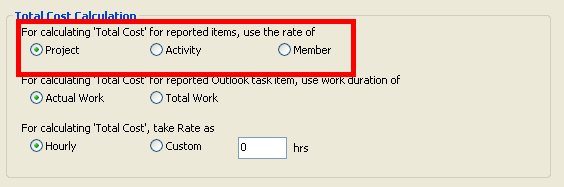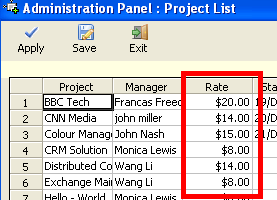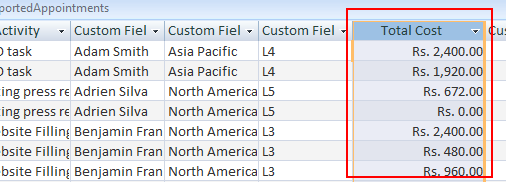# How is total cost calculated for reported timesheet?

You are here:

Total cost of a work would be calculated based on the selected hourly rate of the project, or the Activity or the Team member in a reported timesheet. You can set which hourly rate to use for calculating cost in the reported timesheet from ‘Team TimeSheet Manager > Advanced Options’ admin panel.

For calculating ‘Total Cost’ for reported items, opt either the hourly rate of the Project or Activity or the Member.If project option is chosen, costs are calculated based on the given hourly rate of the project.If Activity option is chosen, costs are calculated based on the given hourly rate of the Activity.If member option is chosen, costs are calculated based on the given hourly rate of the member.So, total cost of the timesheet is computed as:
Total Cost = Chosen Hourly Rate X Duration of the timesheet

Duration for appointment will be the time between the start time and the end time. For tasks, duration will be the work done in minutes as specified under the ‘Total Work’ or ‘Actual Work’ fields of the Outlook task.Once the total cost is computed, the value is fed into the ‘Total Cost’ field of the reported timesheet in the database. (Refer to the screenshot below)